Python Basics
Course

# 8 Pillar Machine Learning Algorithms in Python

9 Lessons Intermediate

About this course

from sklearn.linear_model import LinearRegressionimport numpy as np## Data (Apple stock prices)apple = np.array([155, 156, 157])n = len(apple)## One-linermodel = LinearRegression().fit(np.arange(n).reshape((n,1)), apple)## Result & puzzleprint(model.predict([,]))# What is the output of this code?

This code snippet uses two Python libraries: NumPy and scikit-learn . The former is the de-facto standard library for numerical computations (e.g. matrix operations). The latter is the most comprehensive library for machine learning which implements hundreds of machine learning algorithms and techniques.

So let’s explore the code snippet step by step.

We create a simple dataset of three values: three stock prices of the Apple stock in three consecutive days. The variable ‘apple’ holds this dataset as a one-dimensional NumPy array. We also store the length of the NumPy array in the variable ‘n’.

Let’s say the goal is to predict the stock value of the next two days. Such an algorithm could be useful as a benchmark for algorithmic trading applications (using larger datasets of course).

To achieve this goal, the one-liner uses linear regression and creates a model via the function ‘fit()’. But what exactly is a model?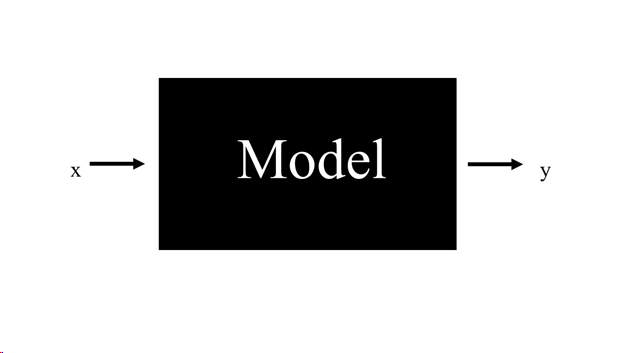Think of a machine learning model as a black box. You put stuff into the box. We call the input “features” and denote them using the variable x which can be a single value or a multi-dimensional vector of values. Then the box does its magic and processes your input. After a bit of time, you get back the result y.

Now, there are two separate phases: the training phase and the inference phase . During the training phase, you tell your model your “dream” output y’. You change the model as long as it does not generate your dream output y’.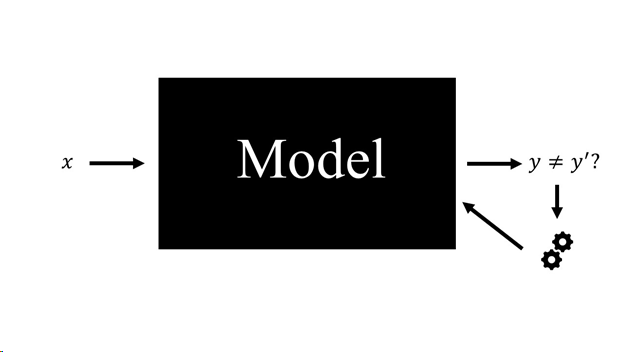As you keep telling the model your “dream” outputs for many different inputs, you “train” the model using your “training data”. Over time, the model will learn which output you would like to get for certain outputs.

That’s why data is so important in the 21st century: your model will only be as good as it’s training data. Without good training data, it is guaranteed to fail.

So why is machine learning such a big deal nowadays? The main reason is that models “generalize”, i.e., they can use their experience from the training data to predict outcomes for completely new inputs which they have never seen before. If the model generalizes well, these outputs can be surprisingly accurate compared to the “real” but unknown outputs.

Now, let’s deconstruct the one-liner which creates the model:

model = LinearRegression().fit(np.arange(n).reshape((n,1)), apple)

First, we create a new “empty” model by calling LinearRegression(). How does this model look like?

Every linear regression model consists of certain parameters. For linear regression, the parameters are called “coefficients” because each parameter is the coefficient in a linear equation combining the different input features.

With this information, we can shed some light into our black box.

Given the input features x_1, x_2, ..., x_k. The linear regression model combines the input features with the coefficients a_1, a_2, ..., a_k to calculate the predicted output y using the formula: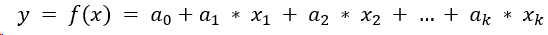In our example, we have only a single input feature x so the formula becomes easier:In other words, our linear regression model describes a line in the two-dimensional space. The first axis describes the input x. The second axis describes the output y. The line describes the (linear) relationship between input and output.What is the training data in this space? In our case, the input of the model simply takes the indices of the days: [0, 1, 2] – one day for each stock price [155, 156, 157]. To put it differently:

• Input x=0 should cause output y=155
• Input x=1 should cause output y=156
• Input x=2 should cause output y=157

Now, which line fits best to our training data [155, 156, 157]?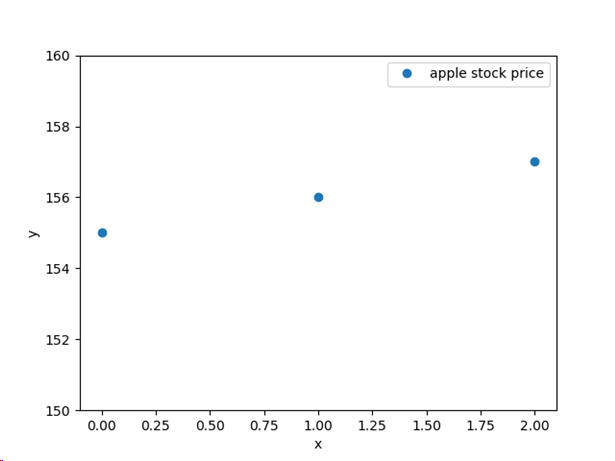Here is what the linear regression model computes:

## Data (Apple stock prices)apple = np.array([155, 156, 157])n = len(apple)## One-linermodel = LinearRegression().fit(np.arange(n).reshape((n,1)), apple)## Resultprint(model.coef_)# [1.]print(model.intercept_)# 155.0

You can see that we have two coefficients: 1.0 and 155.0. Let’s put them in our formula for linear regression: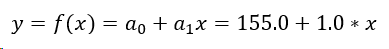Let’s plot both the line and the training data in the same space: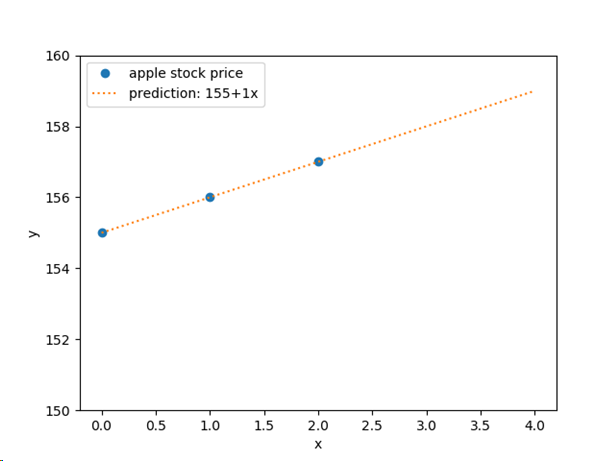A perfect fit! Using this model, we can predict the stock price for any value of x. Of course, whether this prediction accurately reflects the real world is another story.

After having trained the model, we use it to predict the two next days. The Apple dataset consists of three values 155, 156, and 157. We want to know the fourth and fifth value in this series. Thus, we predict the values for indices 3 and 4.

Note that both the function fit() and the function predict() require an array with the following format:

[<training_data_1>, <training_data_2>, …, <training_data_n]

Each training  data value is a sequence of feature value:

<training_data> = [feature_1, feature_2, …,feature_k]

Again, here is our one-liner:

model = LinearRegression().fit(np.arange(n).reshape((n,1)), apple)

In our case, we only have a single feature x. Therefore, we reshape the NumPy array to the strange looking matrix form:

[, , ]

The fit() function takes two arguments: the input features of the training data (see the last paragraph) and the “dream outputs” of these inputs. Of course, our dream outputs are the real stock prices of the Apple stock. The function then repeats testing and tweaking different model parameters (i.e., lines) so that the difference between the predicted model values and the “dream outputs” is minimal. This is called “error minimization”. (To be more precise, the function minimizes the squared difference from the predicted model values and the “dream outputs” so that outliers have a larger impact on the error.)

In our case, the model perfectly fits the training data, so the error is zero. But often it is not possible to find such a linear model. Here is an example of training data that cannot be fit by a single straight line:

from sklearn.linear_model import LinearRegressionimport numpy as npimport matplotlib.pyplot as plt## Data (Apple stock prices)apple = np.array([157, 156, 159])n = len(apple)## One-linermodel = LinearRegression().fit(np.arange(n).reshape((n,1)), apple)## Resultprint(model.predict([,]))# [158. 159.]x = np.arange(5)plt.plot(x[:len(apple)], apple, "o", label="apple stock price")plt.plot(x, model.intercept_ + model.coef_*x, ":", label="prediction")plt.ylabel("y")plt.xlabel("x")plt.ylim((154,164))plt.legend()plt.show()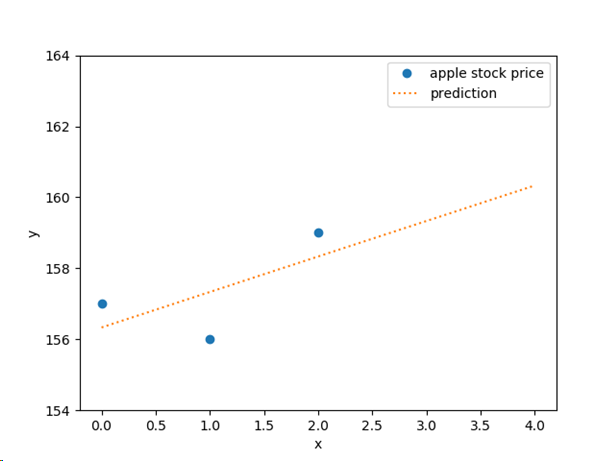In this case, the fit() function finds the line that minimizes the squared error between the training data and the predictions as described above.

FINXTER PREMIUM

FINXTER PREMIUM

FINXTER PREMIUM

FINXTER PREMIUM

FINXTER PREMIUM

FINXTER PREMIUM

FINXTER PREMIUM

FINXTER PREMIUM

FINXTER PREMIUM